Documentation

### This is machine translation

Mouseover text to see original. Click the button below to return to the English version of the page.

Note: This page has been translated by MathWorks. Click here to see
To view all translated materials including this page, select Country from the country navigator on the bottom of this page.

## Banana Function Minimization

This example shows how to minimize Rosenbrock's "banana function":

`$f\left(x\right)=100\left(x\left(2\right)-x\left(1{\right)}^{2}{\right)}^{2}+\left(1-x\left(1\right){\right)}^{2}.$`

$f\left(x\right)$ is called the banana function because of its curvature around the origin. It is notorious in optimization examples because of the slow convergence most methods exhibit when trying to solve this problem.

$f\left(x\right)$ has a unique minimum at the point $x=\left[1,1\right]$ where $f\left(x\right)=0$. This example shows a number of ways to minimize $f\left(x\right)$ starting at the point $x0=\left[-1.9,2\right]$.

### Optimization Without Derivatives

The `fminsearch` function finds a minimum for a problem without constraints. It uses an algorithm that does not estimate any derivatives of the objective function. Rather, it uses a geometric search method described in fminsearch Algorithm.

Minimize the banana function using `fminsearch`. Include an output function to report the sequence of iterations.

```fun = @(x)(100*(x(2) - x(1)^2)^2 + (1 - x(1))^2); options = optimset('OutputFcn',@bananaout,'Display','off'); x0 = [-1.9,2]; [x,fval,eflag,output] = fminsearch(fun,x0,options); title 'Rosenbrock solution via fminsearch'```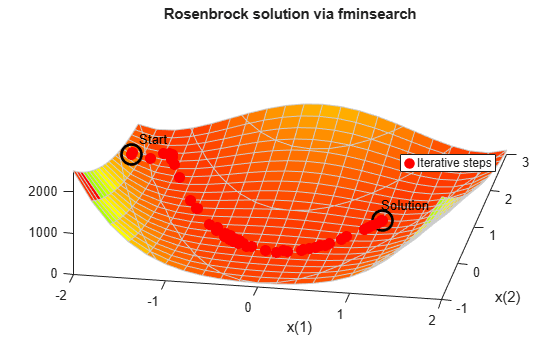```Fcount = output.funcCount; disp(['Number of function evaluations for fminsearch was ',num2str(Fcount)])```
```Number of function evaluations for fminsearch was 210 ```
`disp(['Number of solver iterations for fminsearch was ',num2str(output.iterations)])`
```Number of solver iterations for fminsearch was 114 ```

### Optimization with Estimated Derivatives

The `fminunc` function finds a minimum for a problem without constraints. It uses a derivative-based algorithm. The algorithm attempts to estimate not only the first derivative of the objective function, but also the matrix of second derivatives. `fminunc` is usually more efficient than `fminsearch`.

Minimize the banana function using `fminunc`.

```options = optimoptions('fminunc','Display','off',... 'OutputFcn',@bananaout,'Algorithm','quasi-newton'); [x,fval,eflag,output] = fminunc(fun,x0,options); title 'Rosenbrock solution via fminunc'```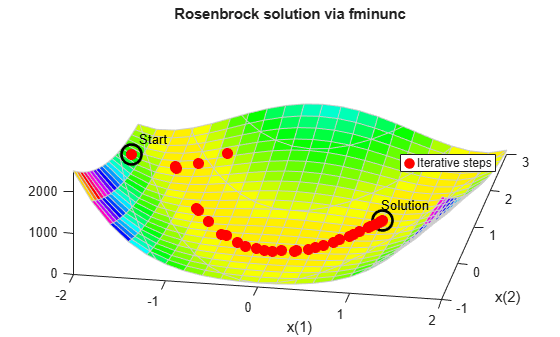```Fcount = output.funcCount; disp(['Number of function evaluations for fminunc was ',num2str(Fcount)])```
```Number of function evaluations for fminunc was 150 ```
`disp(['Number of solver iterations for fminunc was ',num2str(output.iterations)])`
```Number of solver iterations for fminunc was 34 ```

### Optimization with Steepest Descent

If you attempt to minimize the banana function using a steepest descent algorithm, the high curvature of the problem makes the solution process very slow.

You can run `fminunc` with the steepest descent algorithm by setting the hidden `HessUpdate` option to the value `'steepdesc'` for the `'quasi-newton'` algorithm. Set a larger-than-default maximum number of function evaluations, because the solver does not find the solution quickly. In this case, the solver does not find the solution even after 600 function evaluations.

```options = optimoptions(options,'HessUpdate','steepdesc',... 'MaxFunctionEvaluations',600); [x,fval,eflag,output] = fminunc(fun,x0,options); title 'Rosenbrock solution via steepest descent'```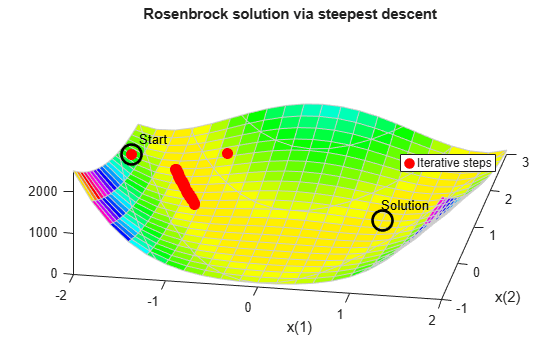```Fcount = output.funcCount; disp(['Number of function evaluations for steepest descent was ',... num2str(Fcount)])```
```Number of function evaluations for steepest descent was 600 ```
```disp(['Number of solver iterations for steepest descent was ',... num2str(output.iterations)])```
```Number of solver iterations for steepest descent was 45 ```

### Optimization with Analytic Gradient

If you provide a gradient, `fminunc` solves the optimization using fewer function evaluations. When you provide a gradient, you can use the `'trust-region'` algorithm, which is often faster and uses less memory than the `'quasi-newton'` algorithm. Reset the `HessUpdate` and `MaxFunctionEvaluations` options to their default values.

```grad = @(x)[-400*(x(2) - x(1)^2)*x(1) - 2*(1 - x(1)); 200*(x(2) - x(1)^2)]; fungrad = @(x)deal(fun(x),grad(x)); options = resetoptions(options,{'HessUpdate','MaxFunctionEvaluations'}); options = optimoptions(options,'SpecifyObjectiveGradient',true,... 'Algorithm','trust-region'); [x,fval,eflag,output] = fminunc(fungrad,x0,options); title 'Rosenbrock solution via fminunc with gradient'```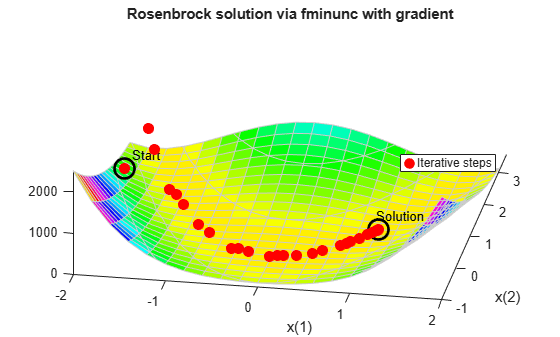```Fcount = output.funcCount; disp(['Number of function evaluations for fminunc with gradient was ',... num2str(Fcount)])```
```Number of function evaluations for fminunc with gradient was 32 ```
```disp(['Number of solver iterations for fminunc with gradient was ',... num2str(output.iterations)])```
```Number of solver iterations for fminunc with gradient was 31 ```

### Optimization with Analytic Hessian

If you provide a Hessian (matrix of second derivatives), `fminunc` can solve the optimization using even fewer function evaluations. For this problem the results are the same with or without the Hessian.

```hess = @(x)[1200*x(1)^2 - 400*x(2) + 2, -400*x(1); -400*x(1), 200]; fungradhess = @(x)deal(fun(x),grad(x),hess(x)); options.HessianFcn = 'objective'; [x,fval,eflag,output] = fminunc(fungradhess,x0,options); title 'Rosenbrock solution via fminunc with Hessian'```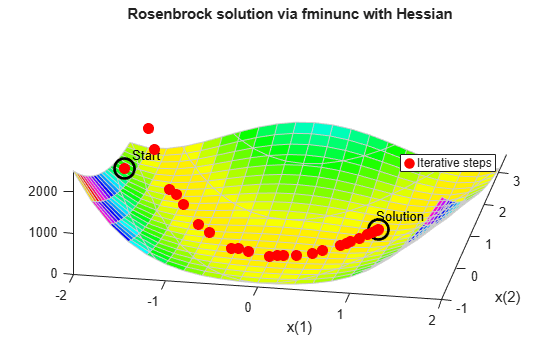```Fcount = output.funcCount; disp(['Number of function evaluations for fminunc with gradient and Hessian was ',... num2str(Fcount)])```
```Number of function evaluations for fminunc with gradient and Hessian was 32 ```
`disp(['Number of solver iterations for fminunc with gradient and Hessian was ',num2str(output.iterations)])`
```Number of solver iterations for fminunc with gradient and Hessian was 31 ```

### Optimization with a Least Squares Solver

The recommended solver for a nonlinear sum of squares is `lsqnonlin`. This solver is even more efficient than `fminunc` without a gradient for this special class of problems. To use `lsqnonlin`, do not write your objective as a sum of squares. Instead, write the underlying vector that `lsqnonlin` internally squares and sums.

```options = optimoptions('lsqnonlin','Display','off','OutputFcn',@bananaout); vfun = @(x)[10*(x(2) - x(1)^2),1 - x(1)]; [x,resnorm,residual,eflag,output] = lsqnonlin(vfun,x0,[],[],options); title 'Rosenbrock solution via lsqnonlin'```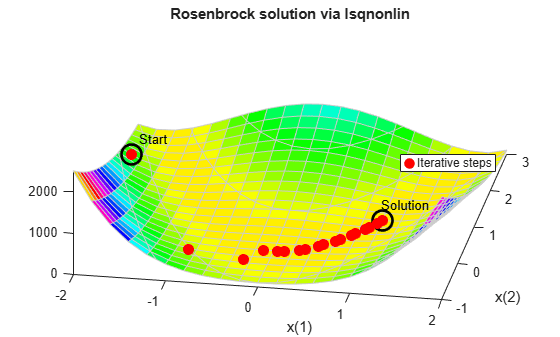```Fcount = output.funcCount; disp(['Number of function evaluations for lsqnonlin was ',... num2str(Fcount)])```
```Number of function evaluations for lsqnonlin was 87 ```
`disp(['Number of solver iterations for lsqnonlin was ',num2str(output.iterations)])`
```Number of solver iterations for lsqnonlin was 28 ```

### Optimization with a Least Squares Solver and Jacobian

As in the minimization using a gradient for `fminunc`, `lsqnonlin` can use derivative information to lower the number of function evaluations. Provide the Jacobian of the nonlinear objective function vector and run the optimization again.

```jac = @(x)[-20*x(1),10; -1,0]; vfunjac = @(x)deal(vfun(x),jac(x)); options.SpecifyObjectiveGradient = true; [x,resnorm,residual,eflag,output] = lsqnonlin(vfunjac,x0,[],[],options); title 'Rosenbrock solution via lsqnonlin with Jacobian'```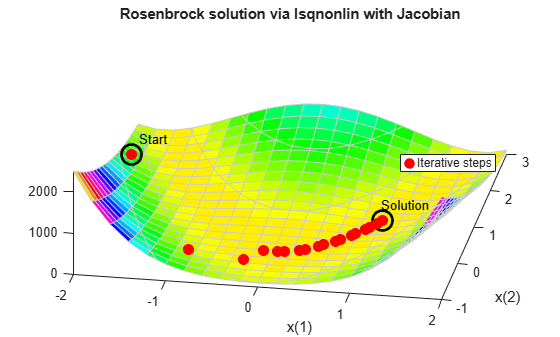```Fcount = output.funcCount; disp(['Number of function evaluations for lsqnonlin with Jacobian was ',... num2str(Fcount)])```
```Number of function evaluations for lsqnonlin with Jacobian was 29 ```
```disp(['Number of solver iterations for lsqnonlin with Jacobian was ',... num2str(output.iterations)])```
```Number of solver iterations for lsqnonlin with Jacobian was 28 ```

Watch now